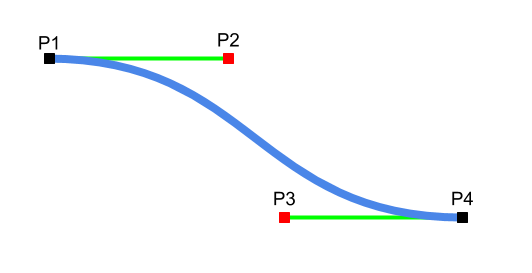Do me a favor, take a few seconds to have a look at my last project.

Thank you, Lulu

# How Bézier curves are described in SVG paths

## Anchor and control points

A section of a Bézier curves is described by 4 points:

• 2 anchor points: also called end point, they describe the coordinates of the starting and final points of the curve ;
• 2 control points: they define the direction of the curve at each anchor points.

In the following illustration, points P1 and P4 are the anchor point. Points P2 and P2 are the control points.

• P2 is the control point associated to the anchor point P1 ;
• P3 is the control point associated to the anchor point P4.## SVG paths : M and C commands

In SVG paths, the commands M and C are used to describe Bézier curves.

• M (move to) is followed by one point (P1) and describe the first anchor point (starting point).
• C (curve) is followed by three points (P2, P3 and P4) and describe the curve (control points and final points)
M x1 y1
C x2 y2     x3 y3       x4 y4

## Examples

The following examples are from w3.org :